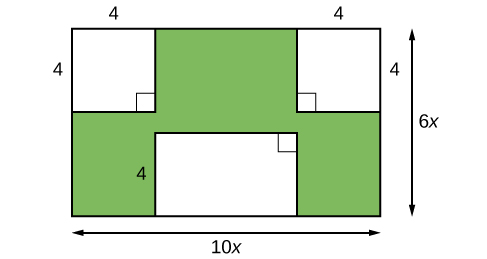## Introduction to Factoring Polynomials

### Learning Objectives

By the end of this section, you will be able to:

• Factor the greatest common factor of a polynomial.
• Factor a trinomial.
• Factor by grouping.
• Factor a perfect square trinomial.
• Factor a difference of squares.
• Factor the sum and difference of cubes.
• Factor expressions using fractional or negative exponents.

Imagine that we are trying to find the area of a lawn so that we can determine how much grass seed to purchase. The lawn is the green portion in Figure 1.Figure 1

The area of the entire region can be found using the formula for the area of a rectangle.

$\begin{array}{ccc}\hfill A& =& lw\hfill \\ & =& 10x\cdot 6x\hfill \\ & =& 60{x}^{2}{\text{ units}}^{2}\hfill \end{array}$

The areas of the portions that do not require grass seed need to be subtracted from the area of the entire region. The two square regions each have an area of $A={s}^{2}={4}^{2}=16$ units2. The other rectangular region has one side of length $10x - 8$ and one side of length $4$, giving an area of $A=lw=4\left(10x - 8\right)=40x - 32$ units2. So the region that must be subtracted has an area of $2\left(16\right)+40x - 32=40x$ units2.

The area of the region that requires grass seed is found by subtracting $60{x}^{2}-40x$ units2. This area can also be expressed in factored form as $20x\left(3x - 2\right)$ units2. We can confirm that this is an equivalent expression by multiplying.

Many polynomial expressions can be written in simpler forms by factoring. In this section, we will look at a variety of methods that can be used to factor polynomial expressions.